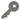# Create Range FansAvailable in real-time and big data analytics.

The Create Range Fans toolgenerates range fans for incoming event record point data using a specified range distance, bearing, and arc angle. A range fan is a two-dimensional feature in the shape of a wedge that can be used to represent a visual or contextual range of a feature.

## Example

A transportation department monitors vehicle movement in real-time and wants to calculate and display the approximate visual range of a moving vehicle. As the vehicle is in transit, the intersection between the vehicle's range fan (field of view) and an asset on the ground can be used to send a notification.

## Usage notes

• The size of the range fan's extent can be set using one of the following options:
• Distance—Uses a constant value (all fans will have the same range extent).
• Field—Uses values from a field (different features can have different-sized range extent).
• Expression—Applies an expression to each feature (different features can have different values based on the expression).
• The range fan's bearing value can be set using one of the following options:
• Value—Uses a constant value (all fans will have the same bearing).
• Field—Uses values from a field (different features can have different bearing values).
• Expression—Applies an expression to each feature (different features can have different values based on the expression).
• The range fan's arc angle can be set using one of the following options:
• Value—Uses a constant value (all range fans will have the same arc angle).
• Field—Uses values from a field (different features can have different arc angle values).
• Expression—Applies an expression to each feature (different features can have different values based on the expression).
• The tool processes an event record to match the spatial reference and coordinate system of the input point features. In other words, the spatial reference of the output polygon feature layer is the same as the input layer.
• If Field is chosen for the Input Layer parameter to obtain the range distances, the field's values can be either a number (5) or a number with a valid linear unit (5 kilometers). If a field value is a number, it is assumed the distance is in the linear unit of the input layer spatial reference (unless the input layer is in a geographic coordinate system, in which case, the value is assumed to be in meters). If the linear unit specified in the field values is invalid or not recognized, the linear unit of the input features spatial reference will be used by default.

## Parameters

ParameterDescriptionData type

Input Layer

The point layer used as the source of the range fan's anchor point.

Features

Range Distance

Specifies a value to calculate the range fan's extent. The range is the length, or distance, the range fan will extend forward of the anchor point. The range can be defined using a constant value, the name of an attribute field, or an expression that evaluates to a positive numeric value. A value is required. The options are as follows:

• Distance—Uses a constant value.
• Field—Uses values from a field.
• If a numeric field is specified, the units will be in the linear unit of the event's spatial reference.
• If a string field is specified with units, the specified units will be used for the range. For example, an event with a field value of 50 miles will create a range fan for that event using 50 miles, regardless of the event's spatial reference.
• Expression—Applies an expression to each feature. The default is Meters. The options are as follows:
• Meters
• Kilometers
• Millimeters
• Centimeters
• Decimeters
• Feet
• Inches
• Yards
• Miles
• Nautical Miles

String; Allowed values: Distance | Field | Expression

Bearing

Specifies the bearing in the number of degrees greater than 0 degrees and less than 360 degrees measured clockwise from north. The bearing can be specified as a constant value, the name of an attribute field, or an expression that evaluates to a positive numeric value. The bearing bisects the range fan's arc. A value is required. The options are as follows:

• Value—Uses a constant value.
• Field—Uses values from a field.
• Expression—Applies an expression to each feature.

String; Allowed values: Value | Field | Expression

Arc Angle

Specifies the arc angle of the range fan in number of degrees greater than 0 degrees and less than 360 degrees. The arc angle can be specified as a constant value, the name of an attribute field, or an expression that evaluates to a positive numeric value. A value is required. The options are as follows:

• Value—Uses a constant value.
• Field—Uses values from a field.
• Expression—Applies an expression to each feature.

String; Allowed values: Value | Field | Expression

## Output layer

The output layer will be a polygon layer and will retain the same schema as the input layer.

## Considerations and limitations

• A range fan can only be constructed forward of a two-dimensional point. Other geometry types such as multipoint, polyline, polygon, and envelope are not supported.
• The Range Distance, Bearing, and Arc Angle parameter values must all evaluate to positive numeric values.
• The Arc Angle parameter value must be greater than 0 degrees and less than 360 degrees. The polygonal range fan may display as a line if an extremely small arc angle is specified (depending on the range, map scale, projection, and feature symbology).